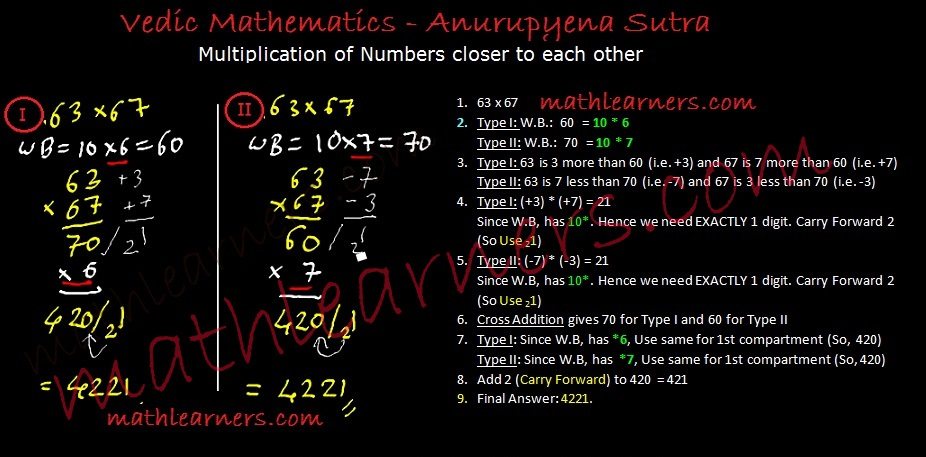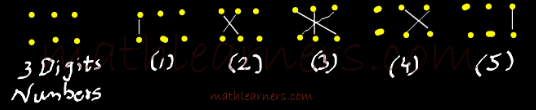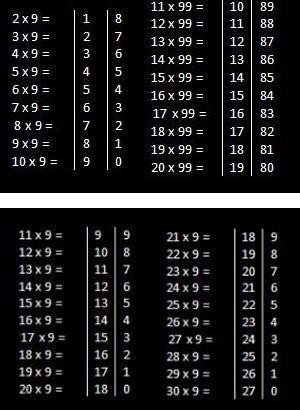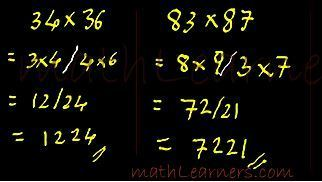educratsweb@mail.com

+91# 5 Best Shortcut Techniques of Multiplication in Vedic Mathematics

Posted By educratsweb.comStudy Material 🗓 Sunday November 17 2019 👁 5

Tirthaji Maharaj has classified tricks to Multiply Numbers in Vedic Mathematics in Specific and General Methods. Specific Multiplication Methods can be applied when numbers satisfy certain conditions like both numbers closer to 100 or numbers closer to each other or addition of last digits of both numbers is 10, etc. While General Multiplication Methods can be applied to any types of numbers.

Depending on Specific and General Techniques, Multiplication in Vedic Mathematics are classified in the form of Sutras as below. Lets see the  Vedic Mathematics Multiplication techniques.

1. Nikhilam Sutra (Specific Technique)
2. Anurupyena Sutra (Specific Technique)
3. Urdhva Tiryak Sutra and Vinculum Process (General Technique)
4. Ekayunena Purvena (Specific Technique)
5. Antyaordaske’pi (Specific Technique)

## Nikhilam Sutra:

This is most simplest trick to multiply numbers using Vedic Mathematics. I personally like this method a lot as multiplication can be done in mind as well.

Using Nikhilam Sutra it is simpler to multiply numbers like 98 & 95, 997 & 987, 102 & 112, 995 & 1008 i.e. the numbers which are closer to power of 10.  This Sutra is a Specific method of Multiplication in Vedic Mathematics which shows shortcuts to multiply numbers which are closer to power of 10 (10, 100, 1000, etc. )

This will generate 3 cases:

• Numbers closer and less than power of 10. Example: 97 * 96, 994 * 992, etc
• Numbers closer and greater than power of 10. Example: 102* 108, 1004 * 1012, etc
• Numbers closer and lying on both sides of power of 10. Example: 102* 95, 1004 * 991, etc

Let’s see few examples on this:Click Here To Check  Process, Types and Examples on Nikhilam Sutra

## Anurupyena Sutra:

This is a sub-type of Nikhilam Sutra and another vedic math multiplication trick when numbers are not closer to power of 10 but are closer to themselves. It works on concept of Working Base and then apply Nikhilam Sutra.

For Example – Multiplication of Numbers like  63 & 67.

Process:

1. Working Base(W.B.) concept: As the numbers (63 & 67) are closer to 60, we take working base as 60 (6*10) instead of 100, here factor is 6.
2. Apply concept of Nikhilam as discussed previously i.e. 63 is 3 greater than 60 and 67 is 7 greater than 60
3. Multiply 3 and 7 to get 21 in 2nd compartment. As base is *10, thus we need to have only 1 digit in 2nd compartment and hence need to carry forward 2 to 1st compartment.
4. Like Nikhilam Sutra, Cross Addition of 63 & 7 or 67 & 3 gives 70.
5. In Anurupyena Sutra, before adding carry forward directly to 1st compartment we need to multiply by the factor (6) and then add the carry forward. This Carry Forward (2) is added to 420
6. Final Answer: 4221

Same multiplication 63 and 67 can be solved by considering Working Base of 70 (10 * 7) as below.Click Here  To understand the Process and More Examples of Anurupyena Sutra

## Urdhva Tiryak Sutra:

This is another great shortcut method of multiplication using Vedic Mathematics. Urdhva Tiryak is General method of multiplication in Vedic Maths which provides shortcut to multiply any types of numbers.

It can be applied very easily to multiply 3 digit numbers, multiply 4 digits numbers and even more than 4 digit numbers.

Lets see an example Multiplication of 3 digit numbers:

Formula Used:  (ax2+bx+c)(dx2+ex+f) = adx4 + (ae+bd)x3 + (af+be+cd)x2 + (bf+ce)x + cf

Process: (Left –> Right)1. Vertical Multiplication of 1st digits of 2 numbers.
2. Crosswise Multiplication Addition of 1st 2 digits 2 numbers. (i.e. Crosswise Multiplication of 1st 2 digits and adding them.)
3. Crosswise Multiplication Addition of all 3 digits of both the numbers.
4. Crosswise Multiplication Addition of last 2 digits 2 numbers.
5. Vertical Multiplication of last digits 2 numbers.
6. For all steps, except 1st step, each compartment needs to have ONLY 1 digits. If not then carry forward initial digits to previous compartment (Check below examples to understand).Click Here to Check Process, Multiplication of 4 & more examples using UrdhvaTiryak Sutra.

## Vinculum Process of Multiplication: �

Vinculum is a special method of Vedic Maths Multiplication which is used with Urdhva Tiryak whenever we have bigger digits like 6,7,8 and 9.

Vinculum is a process applied when numbers have bigger digits like 6,7,8,9. Carrying out operations like multiplication with bigger digits is time consuming and little tougher as compared to smaller digits. Hence such digits 6,7,8 and 9 are converted to smaller digits like 4,3,2 and 1 using Vinculum Process.

I highly recommend to go through the� concept of Vinculum Process.## Ekayunena Purvena Sutra:

This sutra is applicable whenever multiplier has only 9’s as digits.

Example:Click Here => To understand the Process and more examples on Ekayunena Purvena Sutra.

## Antyaordasake’pi:

This sutra has another great multiplication trick in Vedic Mathematics which can be applied when last digits of both numbers totals as 10.

Steps:

1. Check if addition of last digits of the numbers is 10.
2. If yes, multiply them and write in 2nd compartment.
3. Apply Ekadhikena Purvena for the remaining digits i.e. Add 1 to the remaining digits.
4. Eg: In case of 34 x 36, Apply Ekadhikena Purvena on 3 so we have 4. Now multiply 3 and 4 and write in the 1st compartment.if you have any information regarding Job, Study Material or any other information related to career. you can Post your article on our website. Click here to Register & Share your contents.
For Advertisment or any query email us at educratsweb@gmail.com

### RELATED POST

1. Download Study Material for CBSE Class 1 to 8
Download Study Material for CBSE Class 1 to 8 CBSE 1st Class English Medium Textbooks 2021 Pdf Download Marigo
2. वैदिक गणित के 16 सूत्र,उनके अर्थ ,प्रयोग और उदाहरण 16 sutras of vedic mathematics for beginners
वैदिक गणित के 16 सूत्र,  शंकराचार्य स्वामी भारती कृष्ण तीर्थजी महाराज की कृति के बिखरे सन्दर्भों में से अलग किये गए हैं. जिस एक मात्र पुस्तक पर आधारित है वर्तमान में पढ़ा-पढ़ाया जाने वाला वैदिक गणि�
3. 5 Best Shortcut Techniques of Multiplication in Vedic Mathematics
Tirthaji Maharaj has classified tricks to Multiply Numbers in Vedic Mathematics in Specific and General Methods. Specific Multiplication Methods can be applied when numbers satisfy certain conditions like both numbers closer to 100 or numbers closer to each other or addition of last digits of both numbers is 10, etc. While General Multiplication Methods can be applied to any types of numbers. Depending on Specific and Gener
4. 6 Best Shortcut Tricks to divide numbers in Vedic Mathematics
Like Multiplication, Tirthaji Maharaj has classified tricks to Divide Numbers in Vedic Mathematics in Specific and General Methods. Specific Division Methods can be applied when numbers satisfy certain conditions like Divisor slightly less than 100 or Divisor slightly greater than power of 10 or Divisor is ending with 9, etc. While General Multiplication Methods can be applied to any types of numbers. Dependi
5. What is Vedic Mathematics
Vedic Mathematics is a collection of Techniques/Sutras to solve mathematical arithmetics in easy and faster way. It consists of 16 Sutras (Formulae) and 13 sub-sutras (Sub Formulae) which can be used for problems involved in arithmetic, algebra, geometry, calculus, conics. Vedic Mathematics is a system of mathematics which was
6. Tips For Students: How to Take Good Notes for Studying
Taking good notes throughout the semester may mean the difference between simple study sessions or decoding sessions later on. Your time is too valuable for you to just sit in class, day after day, only going through the motions of taking notes. Only writing down what’s on the board won’t cut it. Scribbling incoherently won’t help you either. Try these tips for taking neat class notes that will be of use to you later on for studying and review. Stay focused in class
7. Free Government job study material, old question papers, and mock tests.
Here are some of the freely available Government job study material, old question papers, and mock tests. 1. Andhra Pradesh PSC Mock Test -  https://psc.ap.gov.in/(S(trubzeva4f4b2dcsvnzhln1a))/HomePages/MockTest.aspx 2. Arunachal Pradesh PSC Previous year Question Papers - http://www.appsc.gov.in/PreviousYearQuestionPaper.htm 3. UPSC Model Question Papers and Answers Boo
8. Squares in Vedic Mathematics | Shortcut method for Square any number
Tirthaji Maharaj has mentioned tricks to Square Numbers in Vedic Mathematics in Specific and General Methods. Specific Multiplication Methods can be applied when numbers satisfy certain conditions like number ending with 5 or number closer to power of 10, etc. While General Multiplication Methods can be applied to any type of number. Depending on Specific and General Techniques, Squares in Vedic Mat
We would love to hear your thoughts, concerns or problems with anything so we can improve our website educratsweb.com ! email us at educratsweb@gmail.com and submit your valuable feedback.
Save this page as PDF | Recommend to your Friends5 BEST SHORTCUT TECHNIQUES OF MULTIPLICATION IN VEDIC MATHEMATICS | Google Cached Pages